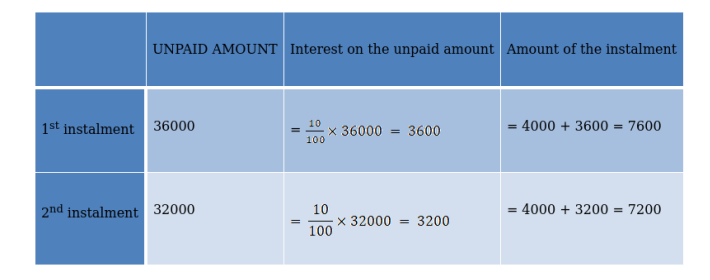# Arun buys a scooter for ₹44000. He pays ₹8000 in cash and agrees to pay

Question:

Arun buys a scooter for ₹44000. He pays ₹8000 in cash and agrees to pay the balance in annual instalments of ₹4000 each plus 10% interest on the unpaid amount. How much did he pay for it?

Solution:

Given:

The amount that is to be paid to buy a scooter = 44000

The amount that he paid by cash = ₹8000

Remaining balance = ₹36000

Annual instalment = ₹4000 + [email protected]% on the unpaid amountThus, our instalments are 7600, 7200, 6800…….

Total number of instalments $=\frac{\text { The remaining balance left }}{\text { balance that is cleared per instalment }}$

$=\frac{36000}{4000}$

= 9

So our instalments are 7600, 7200, 6800 ... up to 9 terms.

Hint: - All our instalments are in A.P with a common difference of 400.

Here

First term, a = 7200

Common difference = d = 7200 - 7600

d = - 400

Number of terms = 9

Sum of all instalments $=s_{n}=\frac{n}{2}\{2 \times a+(n-1) \times d\}$

$=\frac{9}{2}\{2 \times 7600+(9-1) \times(-400)\}$

= 54000

Hence,

The total cost of the scooter = amount that is paid earlier + amount paid in 9 instalments.

= 8000 + 54000

= 62000

∴The total cost paid by Arun = 62000